# Exploring Mathematics in Music

The Fibonacci sequence is a series of numbers that commonly begins with 0 and 1, and every following number of the sequence represents the sum of the previous two. If starting with 0 and 1, the next number in the pattern would be 1, and the following number of the series would be 2. The sequence goes on infinitely, but for the sake of this article we won’t explore past the Fibonacci number of 34. Here’s a longer version of the series.

0, 1, 1, 2, 3, 5, 8, 13, 21, 34, 55, 89, 144, etc.

The Fibonacci sequence has fascinated cultures for centuries. It’s been identified throughout nature and geometry, and many people have applied its pattern to music and visual arts. A modern example can be found in the first five verse sections of the Tool song “Lateralus.” (Note that 5 is a Fibonacci number found in the previous sequence.) Count the vocal’s syllabic groupings while listening to the “Lateralus” verses, and you’ll find Tool front man Maynard James Keenan ascending and descending his way through the sequence within his vocal melody.

A great way to start applying Fibonacci’s series to the drumset is to employ the same concept Keenan used to a bass drum pattern. Exercise 1 applies the numbers 2, 3, 5, and 8 from the Fibonacci sequence as subdivisions (two 8th notes, one triplet, one quintuplet, and eight 32nd notes) in a double bass groove that ascends and descends through those groupings.Closely related to the Fibonacci sequence is the golden ratio. In mathematics, two separate quantities are considered to be in a golden ratio if their ratio to each other is the same as the ratio of their sum to the larger of the two quantities. This relationship can be visualized in the line segment in the following diagram.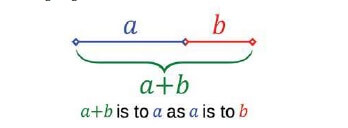Like the Fibonacci sequence, the golden ratio has been used in architecture, painting, music, and other disciplines. Pearl Drums once even based the placement of air vents on a select series of drums on the golden ratio.

To rhythmically relate the golden ratio back to the Fibonacci sequence, we can create what I like to call “golden measures” by using three consecutive Fibonacci numbers. For example, let’s take a measure of 13/8. If we cut that bar into groupings of 8 and 5, we’ve split our measure by approximately the golden ratio, as demonstrated in the following example. We’ll play a measure of 13/16 and voice the bass drum on beat 1 to represent the 8-note grouping, and a snare on beat 3 to represent the 5-note grouping.Exercise 3 explores the golden ratio in a measure of 21/16 with a kick on beat 1 to represent 13 and a snare on the “e” of beat 3 that outlines an 8-note grouping.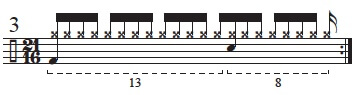The next number in the Fibonacci sequence is 34, which we can express in Exercise 4 with a measure of 17/16 by using 32nd notes that are cut into 21- and 13-note groupings.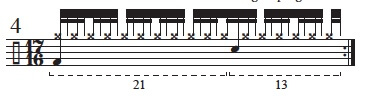The three previous examples each phrased the golden measures with the larger of the two Fibonacci groupings occurring first. However, these rhythms can sound equally interesting in reversed order. In Exercise 5, we’ll attack this idea in a succession of measures that ascend the Fibonacci sequence, alternating from starting each Fibonacci grouping with the bass drum to starting with a snare.Another way we can apply sequential Fibonacci numbers to create “golden rhythms” is within subdivisions. We can explore 8- and 5-note groupings quite effectively in this way. In Exercise 6 we’ll check out a short 2/4 groove that’s constructed of 32nd notes, and we’ll accent a group of 3 notes and a group of 5 notes within each beat.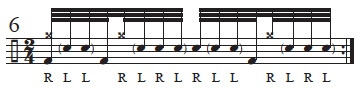In Exercise 7, we’ll try the previous idea in a quintuplet subdivision by accenting a group of 3 and a group of 2 to naturally give us a drunken shuffle feel.If we take the same 8-note rhythm from Exercise 6 and split the 5-note grouping another level deeper into its Fibonacci pair of 3 and 2 on beat 2 of the 2/4 measure, we get an extremely common rhythm, as demonstrated within a 16th-note subdivision in Exercise 8.In Exercise 9 we’ll explore how these rhythms interact by stacking our 8-note bass drum pattern (playing the first, fourth, and seventh partials of the 8-note grouping) underneath a quintuplet subdivision to create another drunken shuffle in 4/4.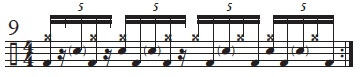Let’s continue to cut the pieces within our golden measures into further Fibonacci splits in a measure of 21/16 by using the same framework that we checked out in Exercise 3. This time, we’ll split 13 into 8- and 5-note groupings, and then we’ll split the grouping of 8 into 5- and 3-note groupings. We’ll begin the second, smaller layers of groupings with a double-stroke on the hi-hats.So far, we’ve mostly voiced each split of the Fibonacci sequence by playing the larger of each grouping’s subsequent division first. (For instance, in Exercise 10, when we split 13 into 8- and 5-note groupings, we played the 8-note grouping first. Likewise in that same exercise, when we split 8 into 5- and 3-note groupings, we played the 5-note grouping first.) In Exercise 11, we’ll build from Exercise 10 but play the smaller of both divided groupings first in that same measure of 21/16. This time we’ll add another bass drum note in our grouping of 13, which is voiced with a 5-note grouping first, and an additional snare in the 8 section, which has a division that begins with a grouping of 3.Notice how in each section this results in another Fibonacci split. In our initial grouping of 13, the 8s have been split into 3- and 5-note groupings, and in the second part of the bar, each grouping of 5 is split further into 2- and 3-note groupings.

Let’s try stacking even more Fibonacci splits into a bar of 4/4. In Exercise 12, we’ll play straight 16th notes on the hi-hats to keep solid time. Our bass drum line descends groupings of 13, 8, 5, 3, 2, and 1 in a 32nd-note subdivision. This will be our initial framework.Finally we’ll add a snare drum and split each of the pieces by one layer deeper of Fibonacci pairs until it collapses in on itself with a final single-note grouping.These represent just a handful of ideas for applying the Fibonacci sequence and golden ratio to rhythms. To learn more about this fascinating set of numbers, check out Mario Livio’s book The Golden Ratio.

Aaron Edgar plays with the Canadian prog-metal band Third Ion and is a session drummer, clinician, and author. His latest book, Progressive Drumming Essentials, is available through Modern Drummer Publications.